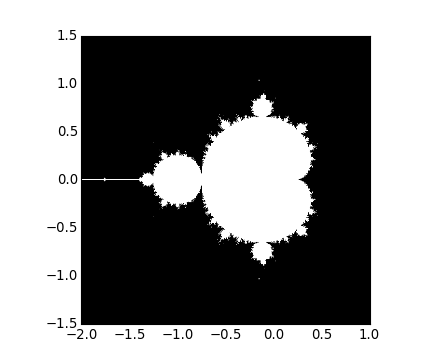# Numpy

NumPy系统是Python的一种开源的数值计算扩展。这种工具可用来存储和处理大型矩阵，比Python自身的嵌套列表（nested list structure)结构要高效的多（该结构也可以用来表示矩阵（matrix））据说NumPy将Python相当于变成一种免费的更强大的MatLab系统。

NumPy（Numeric Python）提供了许多高级的数值编程工具，如：矩阵数据类型、矢量处理，以及精密的运算库。专为进行严格的数字处理而产生。多为很多大型金融公司使用，以及核心的科学计算组织如：Lawrence Livermore，NASA用其处理一些本来使用C++，Fortran或Matlab等所做的任务。

# 多维数组

## 使用numpy.array方法

• 以list或tuple变量为参数产生一维数组：
• 以list或tuple变量为元素产生二维数组：
• 指定数据类型

• 使用numpy.arange方法
• 使用numpy.linspace方法

• 使用numpy.zeros，numpy.ones，numpy.eye

• 创建一个三维数组：

## 获取数组的属性

• 数组索引，切片，赋值
• 使用for操作元素
• 基本的数组运算

• 数组的加减乘除
• 使用数组对象自带的方法
• 使用numpy下的方法
• 合并数组

• 深拷贝数组

• 基本的矩阵运算

• 迹：
• numpy.linalg关于矩阵运算的方法

`>>> import numpy.linalg as nplg`

• 特征值、特征向量：

# 矩阵对象

numpy模块中的矩阵对象为numpy.matrix，包括矩阵数据的处理，矩阵的计算，以及基本的统计功能，转置，可逆性等等，包括对复数的处理，均在matrix对象中。

`class numpy.matrix(data,dtype,copy)`:

• 返回一个矩阵，其中data为ndarray对象或者字符形式；

• dtype:为data的type；

• copy:为bool类型。

## 矩阵对象的属性

• matrix.T transpose

：返回矩阵的转置矩阵

• matrix.H hermitian (conjugate) transpose

：返回复数矩阵的共轭元素矩阵

• matrix.I inverse

：返回矩阵的逆矩阵

• matrix.A base array

：返回矩阵基于的数组

## 矩阵对象的方法

• all([axis, out]) :沿给定的轴判断矩阵所有元素是否为真(非0即为真)

• any([axis, out]) :沿给定轴的方向判断矩阵元素是否为真，只要一个元素为真则为真。

• argmax([axis, out]) :沿给定轴的方向返回最大元素的索引（最大元素的位置）.

• argmin([axis, out]): 沿给定轴的方向返回最小元素的索引（最小元素的位置）

• argsort([axis, kind, order]) :返回排序后的索引矩阵

• astype(dtype[, order, casting, subok, copy]):将该矩阵数据复制，且数据类型为指定的数据类型

• byteswap(inplace) Swap the bytes of the array elements

• choose(choices[, out, mode]) :根据给定的索引得到一个新的数据矩阵（索引从choices给定）

• clip(a_min, a_max[, out]) :返回新的矩阵，比给定元素大的元素为a_max，小的为a_min

• compress(condition[, axis, out]) :返回满足条件的矩阵

• conj() :返回复数的共轭复数

• conjugate() :返回所有复数的共轭复数元素

• copy([order]) :复制一个矩阵并赋给另外一个对象，b=a.copy()

• cumprod([axis, dtype, out]) :返回沿指定轴的元素累积矩阵

• cumsum([axis, dtype, out]) :返回沿指定轴的元素累积和矩阵

• diagonal([offset, axis1, axis2]) :返回矩阵中对角线的数据

• dot(b[, out]) :两个矩阵的点乘

• dumps() :将矩阵的数据转存为字符串.

• fill(value) :将矩阵中的所有元素填充为指定的value

• flatten([order]) :将矩阵转化为一个一维的形式，但是还是matrix对象

• getA() :返回自己，但是作为ndarray返回

• getA1()：返回一个扁平（一维）的数组（ndarray）

• getH() :返回自身的共轭复数转置矩阵

• getI() :返回本身的逆矩阵

• getT() :返回本身的转置矩阵

• max([axis, out]) ：返回指定轴的最大值

• mean([axis, dtype, out]) :沿给定轴方向，返回其均值

• min([axis, out]) ：返回指定轴的最小值

• nonzero() :返回非零元素的索引矩阵

• prod([axis, dtype, out]) :返回指定轴方型上，矩阵元素的乘积.

• ptp([axis, out]) :返回指定轴方向的最大值减去最小值.

• put(indices, values[, mode]) :用给定的value替换矩阵本身给定索引（indices）位置的值

• ravel([order]) :返回一个数组，该数组是一维数组或平数组

• repeat(repeats[, axis]) :重复矩阵中的元素，可以沿指定轴方向重复矩阵元素，repeats为重复次数

• reshape(shape[, order]) :改变矩阵的大小,如：reshape([2,3])

• resize(new_shape[, refcheck]) :改变该数据的尺寸大小

• round([decimals, out]) :返回指定精度后的矩阵，指定的位数采用四舍五入，若为1，则保留一位小数

• searchsorted(v[, side, sorter]) :搜索V在矩阵中的索引位置

• sort([axis, kind, order]) :对矩阵进行排序或者按轴的方向进行排序

• squeeze([axis]) :移除长度为1的轴

• std([axis, dtype, out, ddof]) :沿指定轴的方向，返回元素的标准差.

• sum([axis, dtype, out]) ：沿指定轴的方向，返回其元素的总和

• swapaxes(axis1, axis2):交换两个轴方向上的数据.

• take(indices[, axis, out, mode]) :提取指定索引位置的数据,并以一维数组或者矩阵返回(主要取决axis)

• tofile(fid[, sep, format]) :将矩阵中的数据以二进制写入到文件

• tolist() :将矩阵转化为列表形式

• tostring([order]):将矩阵转化为python的字符串.

• trace([offset, axis1, axis2, dtype, out]):返回对角线元素之和

• transpose(*axes) :返回矩阵的转置矩阵，不改变原有矩阵

• var([axis, dtype, out, ddof]) ：沿指定轴方向，返回矩阵元素的方差

• view([dtype, type]) :生成一个相同数据，但是类型为指定新类型的矩阵。

## 举例